Function Repository Resource:

# DerivativeSignCharts

Get a grid of sign charts for a function and its first and second derivatives

Contributed by: Wolfram|Alpha Math Team
 ResourceFunction["DerivativeSignCharts"][f, x] creates a grid of sign charts for f(x),f'(x) and f''(x).

## Details

DerivativeSignChart accepts all of the options of NumberLinePlot, as well as the following options:
 FontSize Medium adjust the font size of sign chart labels "PlusColor" set the color corresponding to positive points/intervals "MinusColor" set the color corresponding to negative points/intervals "ZeroColor" set the color corresponding to zero or discontinuous points/intervals "ShowPlots" False include plots of the function and its derivatives in the grid "ShowEquations" False include equations of the function and its derivatives in the grid

## Examples

### Basic Examples (1)

Get sign charts for x3 and its first and second derivatives (3x2 and 6x, respectively):

 In:=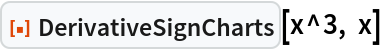Out=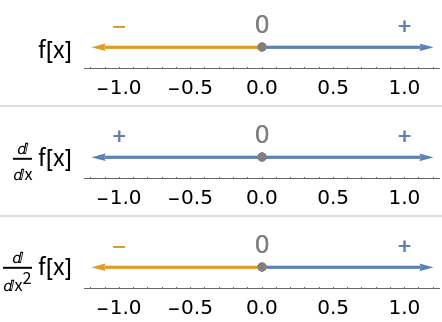### Scope (2)

Include the equations and plots of the given function and its derivatives:

 In:=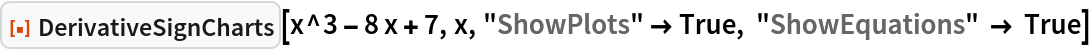Out=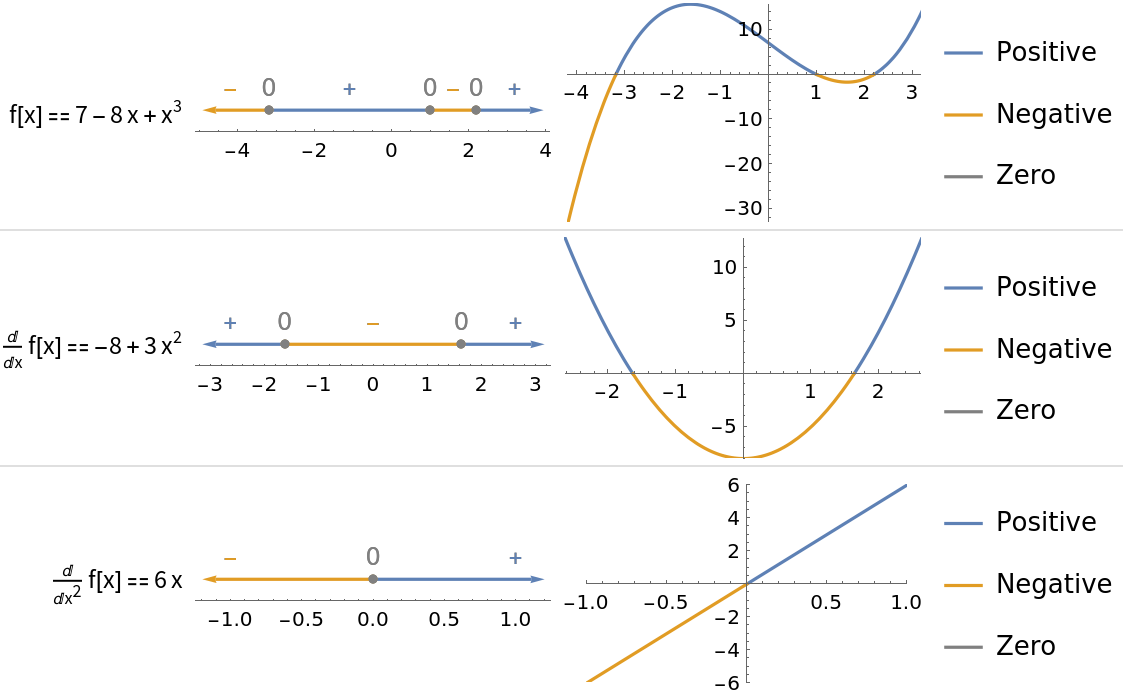If there are infinitely many places where a function or one of its derivatives changes signs, the sign chart displays information for an interval surrounding x = 0:

 In:=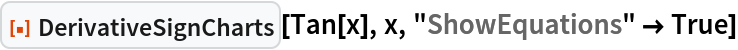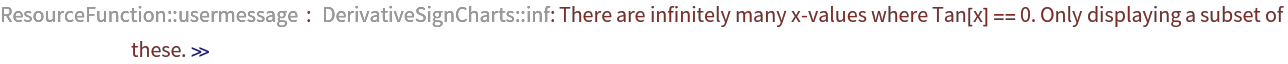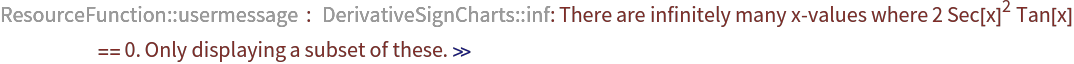Out=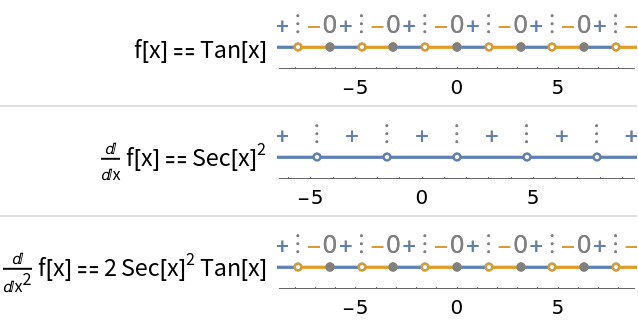### Options (6)

#### FontSize (1)

Use the option FontSize to adjust the size of the sign chart labels:

 In:=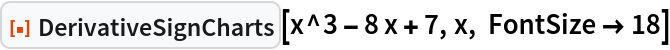Out=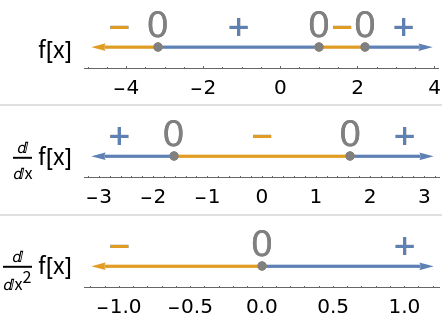#### MinusColor (1)

Use the option "MinusColor" to adjust the color of the negative intervals and minus signs:

 In:=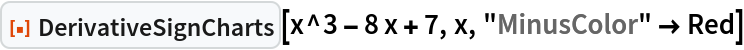Out=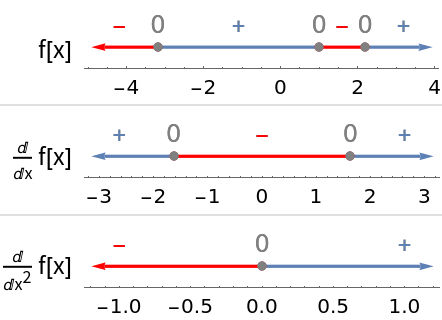#### PlusColor (1)

Use the option "PlusColor" to adjust the color of the positive intervals and plus signs:

 In:=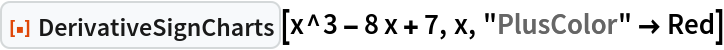Out=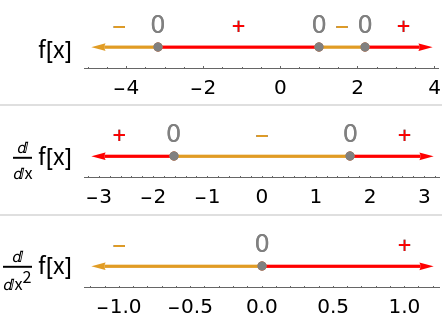#### ZeroColor (1)

Use the option "ZeroColor" to adjust the color of the zero or discontinuous intervals and zeros:

 In:=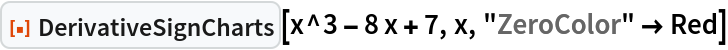Out=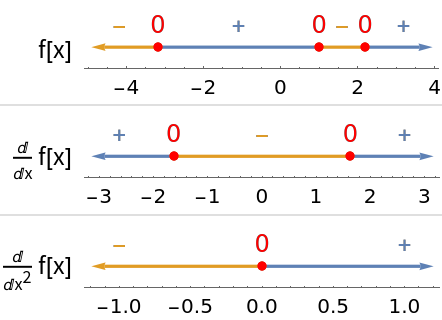#### ShowEquations (1)

Set option “ShowEquations” to True to show the equations of the inputted function and its derivatives:

 In:=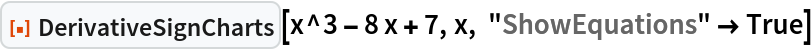Out=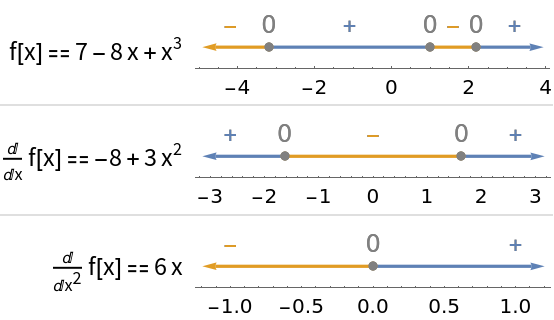#### ShowPlots (1)

Set option “ShowPlots” to True to show plots of the inputted function and its derivatives:

 In:=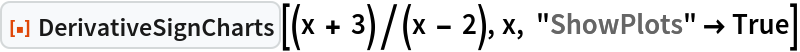Out=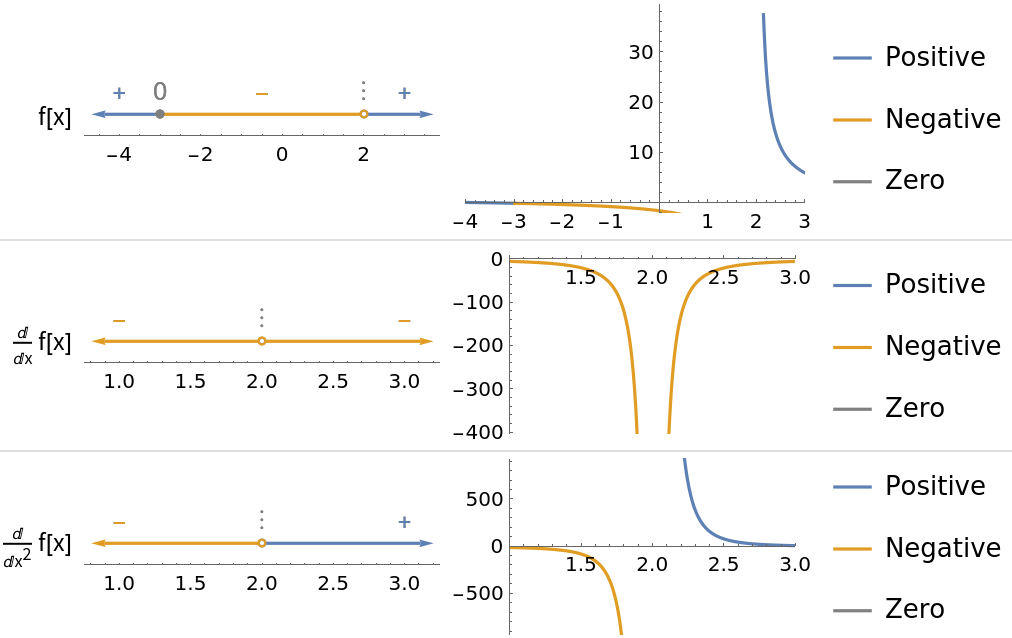### Applications (1)

Sign charts are a useful reasoning tool in many contexts, especially calculus and functional analysis. Comparing the behavior of a function to the signs of its derivatives is foundational to the first and second derivative tests:

 In:=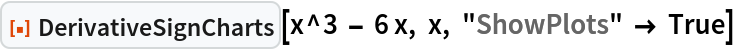Out=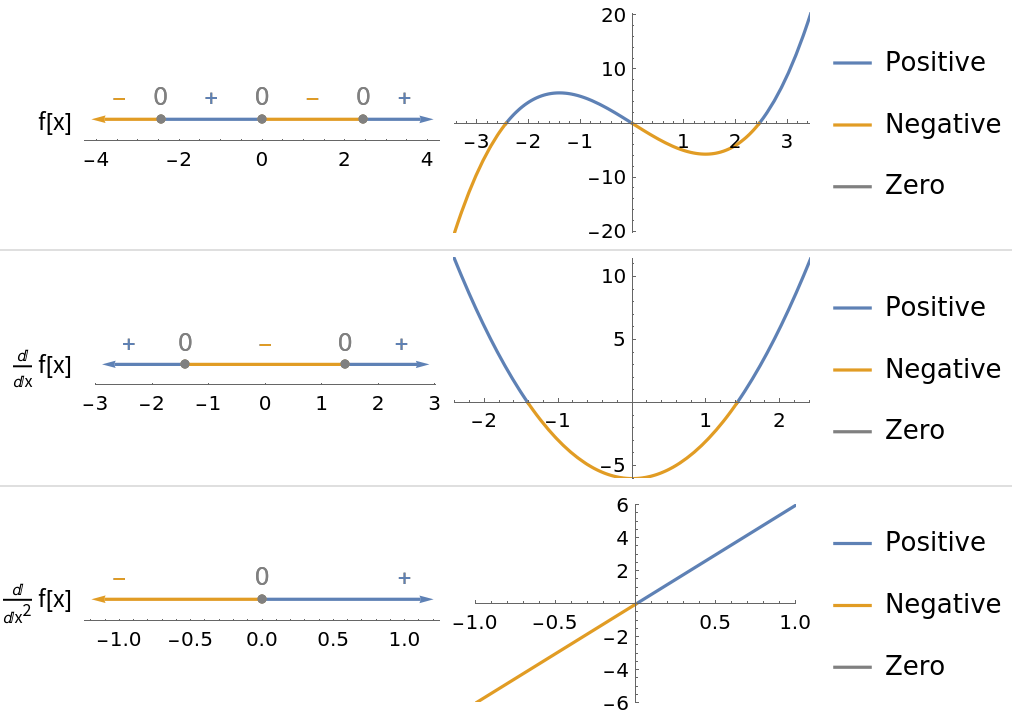## Publisher

Wolfram|Alpha Math Team

## Version History

• 2.0.0 – 23 March 2023
• 1.0.0 – 14 October 2022

## Author Notes

To view the full source code for DerivativeSignCharts, evaluate the following: